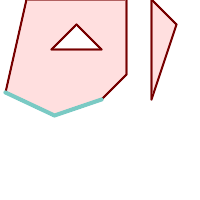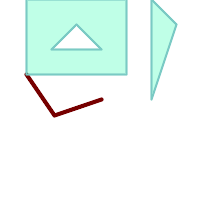## Name

ST_Snap — Snap segments and vertices of input geometry to vertices of a reference geometry.

## Synopsis

`geometry ST_Snap(`geometry input, geometry reference, float tolerance`)`;

## Description

Snaps the vertices and segments of a geometry to another Geometry's vertices. A snap distance tolerance is used to control where snapping is performed. The result geometry is the input geometry with the vertices snapped. If no snapping occurs then the input geometry is returned unchanged.

Snapping one geometry to another can improve robustness for overlay operations by eliminating nearly-coincident edges (which cause problems during noding and intersection calculation).

Too much snapping can result in invalid topology being created, so the number and location of snapped vertices is decided using heuristics to determine when it is safe to snap. This can result in some potential snaps being omitted, however.The returned geometry might lose its simplicity (see ST_IsSimple) and validity (see ST_IsValid).

Performed by the GEOS module.

Availability: 2.0.0

## ExamplesA multipolygon shown with a linestring (before any snapping)A multipolygon snapped to linestring to tolerance: 1.01 of distance. The new multipolygon is shown with reference linestring ```SELECT ST_AsText(ST_Snap(poly,line, ST_Distance(poly,line)*1.01)) AS polysnapped FROM (SELECT ST_GeomFromText('MULTIPOLYGON( ((26 125, 26 200, 126 200, 126 125, 26 125 ), ( 51 150, 101 150, 76 175, 51 150 )), (( 151 100, 151 200, 176 175, 151 100 )))') As poly, ST_GeomFromText('LINESTRING (5 107, 54 84, 101 100)') As line ) As foo; polysnapped --------------------------------------------------------------------- MULTIPOLYGON(((26 125,26 200,126 200,126 125,101 100,26 125), (51 150,101 150,76 175,51 150)),((151 100,151 200,176 175,151 100))) ```A multipolygon snapped to linestring to tolerance: 1.25 of distance. The new multipolygon is shown with reference linestring ```SELECT ST_AsText( ST_Snap(poly,line, ST_Distance(poly,line)*1.25) ) AS polysnapped FROM (SELECT ST_GeomFromText('MULTIPOLYGON( (( 26 125, 26 200, 126 200, 126 125, 26 125 ), ( 51 150, 101 150, 76 175, 51 150 )), (( 151 100, 151 200, 176 175, 151 100 )))') As poly, ST_GeomFromText('LINESTRING (5 107, 54 84, 101 100)') As line ) As foo; polysnapped --------------------------------------------------------------------- MULTIPOLYGON(((5 107,26 200,126 200,126 125,101 100,54 84,5 107), (51 150,101 150,76 175,51 150)),((151 100,151 200,176 175,151 100))) ```The linestring snapped to the original multipolygon at tolerance 1.01 of distance. The new linestring is shown with reference multipolygon ```SELECT ST_AsText( ST_Snap(line, poly, ST_Distance(poly,line)*1.01) ) AS linesnapped FROM (SELECT ST_GeomFromText('MULTIPOLYGON( ((26 125, 26 200, 126 200, 126 125, 26 125), (51 150, 101 150, 76 175, 51 150 )), ((151 100, 151 200, 176 175, 151 100)))') As poly, ST_GeomFromText('LINESTRING (5 107, 54 84, 101 100)') As line ) As foo; linesnapped ---------------------------------------- LINESTRING(5 107,26 125,54 84,101 100) ```The linestring snapped to the original multipolygon at tolerance 1.25 of distance. The new linestring is shown with reference multipolygon ```SELECT ST_AsText( ST_Snap(line, poly, ST_Distance(poly,line)*1.25) ) AS linesnapped FROM (SELECT ST_GeomFromText('MULTIPOLYGON( (( 26 125, 26 200, 126 200, 126 125, 26 125 ), (51 150, 101 150, 76 175, 51 150 )), ((151 100, 151 200, 176 175, 151 100 )))') As poly, ST_GeomFromText('LINESTRING (5 107, 54 84, 101 100)') As line ) As foo; linesnapped --------------------------------------- LINESTRING(26 125,54 84,101 100) ```